Pressure versus temperature graph of an ideal gas is as shown in figure. Density of the gas at point A is ${\mathrm{\rho }}_{0}$ . Density at B will be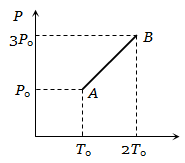1.  $\frac{3}{4}{\mathrm{\rho }}_{0}$

2.  $\frac{3}{2}{\mathrm{\rho }}_{0}$

3.  $\frac{4}{3}{\mathrm{\rho }}_{0}$

4.

High Yielding Test Series + Question Bank - NEET 2020

Difficulty Level:

The figure shows graphs of pressure versus density for an ideal gas at two temperatures ${\mathrm{T}}_{1}$ and ${\mathrm{T}}_{2}$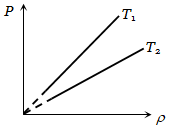1. ${\mathrm{T}}_{1}>{\mathrm{T}}_{2}$

2. ${\mathrm{T}}_{1}={\mathrm{T}}_{2}$

3. ${\mathrm{T}}_{1}<{\mathrm{T}}_{2}$

4. Nothing can be predicted

High Yielding Test Series + Question Bank - NEET 2020

Difficulty Level:

Graph of specific heat at constant volume for a monoatomic gas is

1.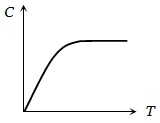2.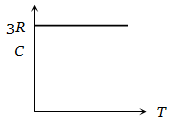3.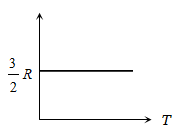4.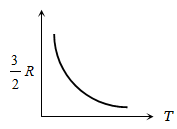High Yielding Test Series + Question Bank - NEET 2020

Difficulty Level:

A fix amount of nitrogen gas (1 mole) is taken and is subjected to pressure and temperature variation. The experiment is performed at high pressure as well as high temperatures. The results obtained are shown in the figures. The correct variation of PV/RT with P will be exhibited by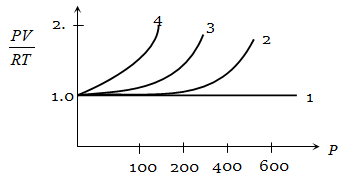1.  4

2.  3

3.  2

4.  1

High Yielding Test Series + Question Bank - NEET 2020

Difficulty Level:

A pressure P - absolute temperature T diagram was obtained when a given mass of gas was heated. During the heating process from the state 1 to state 2 the volume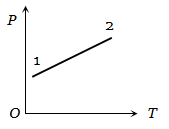1. Remained constant

2. Decreased

3. Increased

4. Changed erratically

High Yielding Test Series + Question Bank - NEET 2020

Difficulty Level:

A volume V and pressure P diagram was obtained from state 1 to state 2 when a given mass of a gas is subjected to temperature changes. During this process the gas is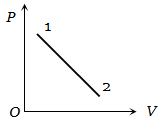1. Heated continuously

2. Cooled continuously

3. Heated in the beginning and cooled towards the end

4. Cooled in the beginning and heated towards the end

High Yielding Test Series + Question Bank - NEET 2020

Difficulty Level:

The figure shows the volume V versus temperature T graphs for a certain mass of a perfect gas at two constant pressures of ${\mathrm{P}}_{1}$ and ${\mathrm{P}}_{2}$ . What interference can you draw from the graphs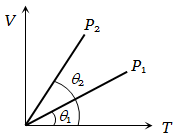1.

2.

3.  ${\mathrm{P}}_{1}={\mathrm{P}}_{2}$

4. No interference can be drawn due to insufficient information

High Yielding Test Series + Question Bank - NEET 2020

Difficulty Level:

The change in volume V with respect to an increase in pressure P has been shown in the figure for a non-ideal gas at four different temperatures  and ${\mathrm{T}}_{4}$ . The critical temperature of the gas is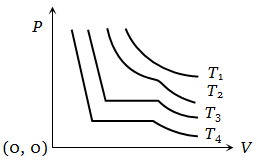1. ${\mathrm{T}}_{1}$

2. ${\mathrm{T}}_{2}$

3. ${\mathrm{T}}_{3}$

4. ${\mathrm{T}}_{4}$

High Yielding Test Series + Question Bank - NEET 2020

Difficulty Level:

In the adjoining figure, various isothermals are shown for a real gas. Then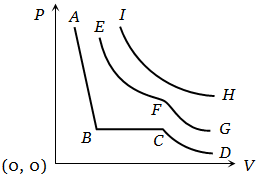1. EF represents liquification

2. CB represents liquification

3. HI represents the critical temperature

4. AB represents gas at a high temperature

Difficulty Level:

From the following P-T graph what interference can be drawn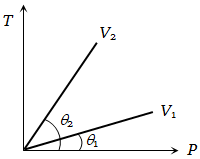1.

2.

3.

4. None of the above